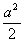# A circle of maximum possible size is cut from a square sheet of board. Subsequently, a square of maximum possible size is cut from the resultant circle. What will be area of the final square?

14 years ago

This question is quite simple......

Let the side of the original square be 'a' units. Therefore, the area of the original square = a2 units.

The diameter of the circle of maximum possible dimension that is cut from the square will be 'a' units.

The diagonal of the square of maximum possible dimension that can be cut from the circle will be 'a' units.

If the diagonal of the final square is 'a' units, then its area =units.
Therefore, the area of the new square will be 50% of the area of the original square.